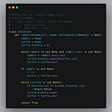# Regular Expression Matching

Hi! Today we are going to talk about a very useful concept known as regular expressions also called regex.

## What is regex?

Regex is basically a pattern that can represent a variety of strings. In regex vocabulary, few characters carry a special meaning. Two of those that are relevant to the current post are:

. in regex -> can match any single character
.* in regex -> can match zero or more characters preceding *

Regex is used everywhere. For example, regex is used in search engines. In websites also, where users are required to enter their email address, you can validate the field using a regular expression that matches the structure of an email address. Regex has many use cases in a string validation and string searching.

Suppose our pattern is, p= aa.c and the search string is, s=aabc
We can search this string with our pattern, or we can say that this pattern can represent s. Here . can represent a single character, so b can be represented using dot character. This pattern can also represent other strings like, aacc, aayc, and so on. Similarly, for the patterns like a.*b, they can effectively represent strings like “ab”, “acb”, “accb”, “actb”, “ahhkb”, and so on.

## Let’s understand how we can implement an algorithm for achieving the target of regular expression matching. Here is our problem statement.

Problem Statement: Given an input string (`s`) and a pattern (`p`), implement regular expression matching with support for `'.'` and `'*'`.

'.' Matches any single character.
'*' Matches zero or more of the preceding element.

The matching should cover the entire input string (not partial).

Before we begin, please make sure you read the below assumptions we are making to go about solving this problem.

There are a few cases to consider. For all the cases, we will be iterating our strings. Here, p will represent our pattern string and s will represent our input string. The character i will denote the index of the input string and j will denote the index of our pattern string. Initially, i and j represent the last characters of both the strings.

There are basically three cases that we need to consider. Without further delay, let’s see them all.

# CASE 1: Last character of both the strings matches.

s[i] == p[j] or p[j] == ‘.’

Here, s can be represented by p, if, the last character of both s and p matches and the substring of s and p, before the last character, also matches. Basically, the prefix of s and p are matching.

Here, s[i] == p[j], we need to check whether, s[0..i-1] can be represented by p[0..j-1]. In this case, ab can be formed using ab. That means s can be formed using p. Therefore, we checked for smaller subproblems. We can break a bigger problem into smaller subproblems. Let’s consider another case.

Here, s[i] != p[j], but p[j] == ‘.’ and by the definition of regex, ‘.’ can represent any single character. Therefore, we can say, ‘d’ can be represented using ‘.’. Now we need to check for smaller subproblem. Check whether s[0..i-1] can be represented by p[0..j-1]. In this case, ab can be formed using ab. That means s can be formed using p.

Let’s see another case. Here, s[i] == p[j]. Now we need to check for smaller subproblem. Check whether s[0..i-1] can be represented by p[0..j-1]. In this case, ab can not be formed using string cb. That means s can not be formed using p.

This suggests that when the last characters of s and p matches, the result of smaller subproblems will be our result. That is, we are recursively checking for the smaller subproblem.

# CASE 2: Last character pattern string is *

It has two cases. Either the character preceding * is occurring once or more than once or it is not occurring at all.

## Character preceding * occurring once or more than once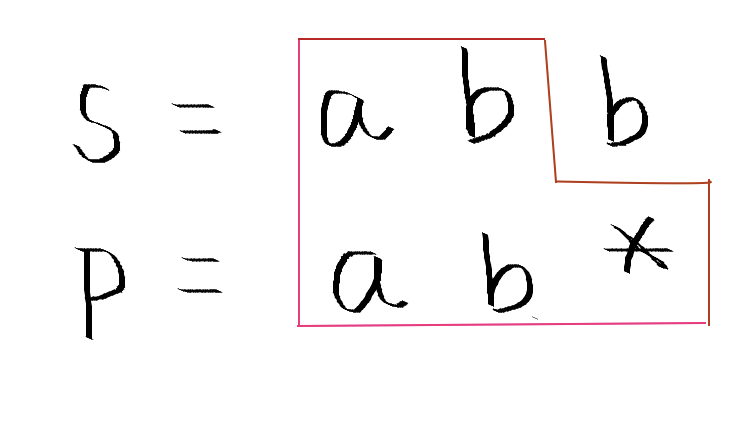More than one occurrence of the character preceding * in the input string

If the last character in the pattern is *. It means there can be either zero or more occurrence of character before * in the input string. We will check if the character before * matches with the current character, i.e. whether s[i] == p[j-1] or p[j-1]==’.’. The characters match, as b matches with b, check for the result of the smaller subproblem. That is, by removing the last character from the input string. Check for s[0..i-1] and p[0..j]. As we can see ab matches with ab*, therefore s can be generated using p. Let’s see for the smaller problem just generated.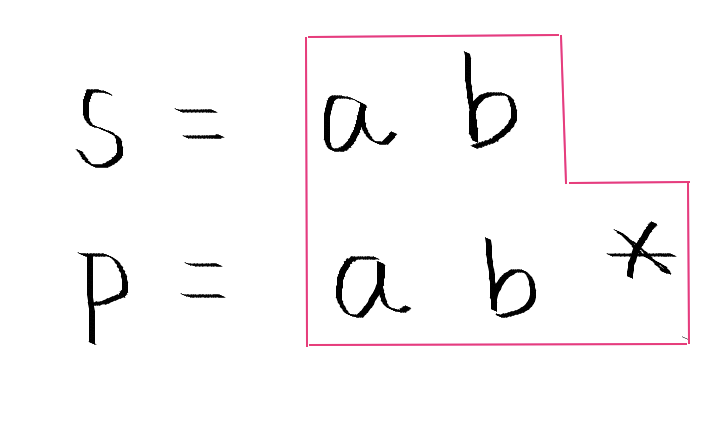One occurrence of the character preceding * in the input string

We are left with this input string s after breaking it into smaller sub-problem. Here again, we can see that the character preceding the last character of * matches with the current character of the input string, i.e. b matches with b (s[i] == p[j-1] || or p[j-1]==’.’). We again need to break the problem into smaller subproblems. Remove the last character of the input string and again try to match.

## Character preceding * not present in the input string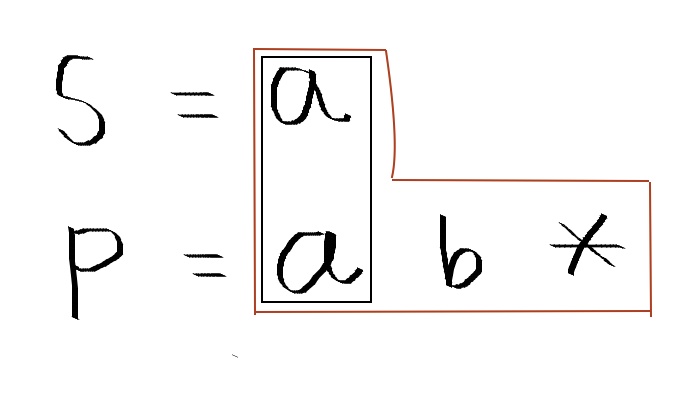Zero occurrences of the character preceding * in the input string

We are left with this input string s after breaking it into smaller sub-problem. By the definition of regex, we know that character before * may not occur in the input string even once as well. Therefore match the current character of the input string with the character before the preceding character of * in the pattern string. That is, match s[i] with p[j-2] or if p[j-2]=’.’ . We can see that s[i]=a and p[j-2]=a. We have just broken down the problem as matching of string s[0..i] and p[0..j-2]. These smaller subproblems match. Therefore, the final result is, s can be formed using p.

# CASE 3: Last character pattern string does not match the last character of the input string and it is not * or .

In this case, we can’t break the problem into smaller subproblems as the last characters don’t match. Therefore, simply return that the strings can not match.

These are the basic cases involved in this problem. As it is a recursive algorithm there must be a base case as well to stop the recursion at some point.

You can actually reach the base condition yourself. Try to figure out yourself first and then continue here.

## BASE CONDITION

1. When both the input string and pattern becomes null, then that actually means pattern can represent input string, return true in that case. (i<0 and j<0)
2. When the pattern is an empty string and input string is non-empty, then we can not generate input string using the pattern string, return false in this case. (i>0 and j==0)
3. This one is a tricky base case now. When the input string is empty and the pattern is non-empty. We can still generate the input string using the pattern. Let’s see how.

Suppose pattern = a*b*
and input = “”
As you can see, pattern means, zero or more times a followed by zero or more times b. Therefore, strings that can be generated by the pattern are: “”, “a”, “ab”, “aab”, “aa”, “abb”, “b”, “bb”, and so on.

Suppose in our recursive call, the input string is empty, therefore i=-1. And in the pattern string j=0, we see that we can’t generate empty string using “a”. But at j=1, we see that we can generate empty string using “a*”. Similarly, at j=3 we can generate empty string using “a*b*”. So our third base case will be,

if(i<0 && p[j]==’*’) regex(s,p, i, j-2);

if(i<0 && p[j]!=’*’) return false;

So, these are the three base cases for recursion. I hope the solution is clear to you. Try to create a recursive function using the concepts explained above.

## The recursive solution, Top-down approach

`boolean regex(s, p, i, j){ //base conditions if(i<0 && j<0) return true; if(j<0 || (i<0 && p[j]!='*')) return false; if(i<0 && p[j]=='*') return regex(s,p,i,j-2); if(s[i]==p[j] || p[j]=='.') return regex(s,p,i-1,j-1); if(p[j]=='*'){   boolean result = false;  if(s[i]==p[j-1] || p[j]=='.'){    result = regex(s,p,i-1,j)  }  if(!result){    return (result || regex(s,p,i,j-2));  } } return false;}`

As in a recursive function, there are many instances where a subproblem is calculated multiple times. In the recursive call, time complexity will be exponential. We need to memoize the solution and reduce the time complexity. For that, we can use the concept of dynamic programming.

## Memoization and Bottom-up approach

Our DP array size will be (s.length()+1) x (p.length()+1)
This is a boolean array, where s[i][j] will store whether it’s possible to generate string s[0..i] using pattern p[0..j]. I am filling this DP array in a bottom-up manner.

The first row and first column of the DP will correspond to the empty input string and empty pattern string respectively. You can fill the first row and first column of the boolean DP array using the base conditions explained above.

Considering the size of the input string as m and size of pattern string as n.

This solution has a space complexity of O((m+1) x (n+1)). Along with that it has a time complexity of O((m+1) x (n+1)).

Hurrah! We are done! Thanks for devoting your time to this article. I hope you find it helpful and it gives you a clear insight into the topic.

If you have any doubts, feel free to ask in the comment section below. If you like this blog, please share it with your friends. Your claps motivate me for writing more such blogs.

Stay tuned for the upcoming explanatory posts by following me on medium.

Have a good day and enjoy coding!! :D

--

--

--

## More from TRICK THE INTERVIEWER

Programming interview is the one where the interviewer will make all possible attempts to trick you. Trick the interviewer this time! :)

## JavaScript execution context part 2 — call stack and multiple execution contexts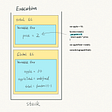## React-Native Halloween fiddle using RN Animated API## How to Customizing Laravel’s default notification email template## Making of my first cross-platform app with React Native## Learn Web3 in 100 Days — Day 11: Front-End Framework## Questions, Questions, Questions## Write Your Own Vanilla SPA Framework## Jhanak Didwania

Software Engineer | Programmer

## Leetcode problem 1249: Minimum Remove to Make Valid Parentheses## 125. Valid Palindrome in Python3